§15.19. Arithmetic with units

The example equations in the previous section carried out quite a lot of arithmetic, but they may have given the impression that Inform always allows arithmetic - which is not true.

This is actually a good thing, because it keeps us from error. For instance, Inform will not allow:

Equation - Newton's Totally Bogus Law
F = m^2
where F is a force, m is a mass.

because whatever you get when you square a mass, you don't get a force - in the same way that a length times another length makes an area, not another length. Physicists call this "dimensional analysis", and it often provides clues about which equations are right. Just after the Second World War, someone correctly worked out the explosive power of an atomic bomb without any classified information simply by guessing what values would appear in the formula, and then finding the simplest equation they could appear in.

In general, Inform will not allow numerical kinds of value to be multiplied or divided by each other (or square or cube rooted) unless we give it instructions that this would make sense.

Of course, there's plenty we can still do without any need for such instructions. For instance, going back to weight,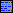The Weighbridge is a room. "A sign declares that the maximum load is [100kg multiplied by 3]."

...will produce the text "A sign declares that the maximum load is 300kg." Here Inform knows that it makes sense to multiply a weight by 3, and that the result will be a weight. Similarly, Inform allows us to add and subtract weights, and several different forms of division are allowed:The blackboard is in the Weighbridge. "A blackboard propped against one wall reads: '122 / 10 is [122 divided by 10] remainder [remainder after dividing 122 by 10]; but 122kg / 10kg is [122kg divided by 10kg] remainder [remainder after dividing 122kg by 10kg]; and 122kg / 10 is [122kg divided by 10] remainder [remainder after dividing 122kg by 10].'"

When we visit the Weighbridge, we find:

A blackboard propped against one wall reads: "122 / 10 is 12 remainder 2; but 122kg / 10kg is 12 remainder 2kg; and 122kg / 10 is 12kg remainder 2kg."

Whereas we are not allowed to divide 122 by 10kg: that would make no sense, since 122 is a number and not made up of kilograms. Inform will produce a problem message if we try. Similarly, Inform won't normally allow us to multiply two weights together - but see the next section.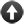Start of Chapter 15: Numbers and Equations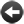Back to §15.18. Equations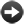Onward to §15.20. Multiplication of units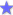ExampleFrozen Assets A treatment of money which keeps track of how much the player has on him, and a BUY command which lets him go shopping.ExampleMoney for Nothing An OFFER price FOR command, allowing the player to bargain with a flexible seller.ExampleLemonade Containers for liquid which keep track of how much liquid they are holding and of what kind, and allow quantities to be moved from one container to another.ExampleSavannah Using the liquid implementation demonstrated in Lemonade for putting out fires.